Complex Numbers
Question: Does the equation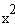=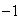have a solution?
Certainly not in the real number system, but we can create a larger number
system by adding a special number i defined as:=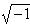(we call i the imaginary unit)
Then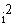=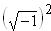=Also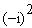=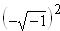=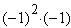=Thus both i and -i are solutions to the equation given above.
Remember that=Definition: A complex number has the form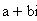where a and b are real numbers. This is the standard form of a complex number.
Also, a complex number a+bi is called an imaginary number if b is not zero.
If a is zero, then the complex number reduces to bi and is called a pure
imaginary number.
Definition: The conjugate ofis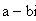Definitions of equality, addition, and multiplication:=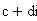means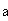=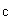and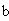=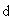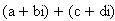=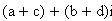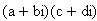=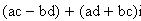Note: People do not usually use this complicated definition of multiplication.
Instead, they multiply using the usual First, Outer, Inner, Last pattern and then use=Example: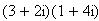=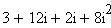=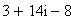=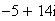Theorem 1: A useful shortcut to remember is that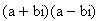=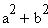Properties:
1) Addition and multiplication are commutative:=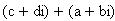=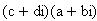2) Addition and multiplication are associative: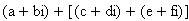=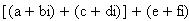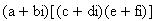=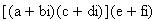3)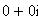=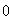is the additive identity: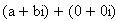=4)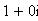=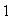is the multiplicative identity: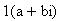=5) Every complex numberhas additive inverse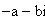6) Every nonzero complex numberhas a multiplicative inverse: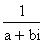=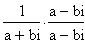=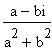=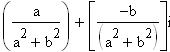7) Multiplication distributes over addition: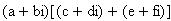=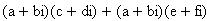Definitions of negation, subtraction, and division: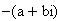=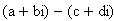=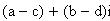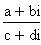=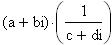where we assume that c+di is not zero and
can thus find the reciprocal as above
Definition: The principal square root of a negative real number is defined by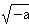=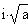where a > 0
The other square root of -a is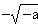=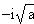Warning: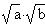=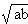holds for non-negative reals a and b, but it may
fail to hold in other cases. To be precise, it fails when both a and b are
negative.
Example: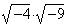compared with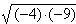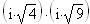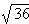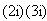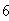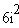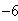We do not get the same answer.